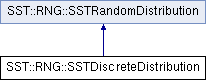SST  6.0.0 StructuralSimulationToolkit
SST::RNG::SSTDiscreteDistribution Class Reference

Creates a discrete distribution for use within SST. More...

`#include "sst/core/rng/discrete.h"`

Inheritance diagram for SST::RNG::SSTDiscreteDistribution:## Public Member Functions

SSTDiscreteDistribution (const double *probs, const uint32_t probsCount)
Creates an exponential distribution with a specific lambda. More...

SSTDiscreteDistribution (const double *probs, const uint32_t probsCount, SSTRandom *baseDist)
Creates an exponential distribution with a specific lambda and a base random number generator. More...

~SSTDiscreteDistribution ()
Destroys the exponential distribution.

double getNextDouble ()
Gets the next (random) double value in the distribution. More...Public Member Functions inherited from SST::RNG::SSTRandomDistribution
virtual ~SSTRandomDistribution ()
Destroys the distribution.

SSTRandomDistribution ()
Creates the base (abstract) class of a distribution.

## Protected Attributes

SSTRandombaseDistrib
Sets the base random number generator for the distribution.

bool deleteDistrib
Controls whether the base distribution should be deleted when this class is destructed.

double * probabilities
The discrete probability list.

uint32_t probCount
Count of discrete probabilities.

## Detailed Description

Creates a discrete distribution for use within SST.

This distribution is the same across platforms and compilers.

## Constructor & Destructor Documentation

 SST::RNG::SSTDiscreteDistribution::SSTDiscreteDistribution ( const double * probs, const uint32_t probsCount )
inline

Creates an exponential distribution with a specific lambda.

Parameters
 lambda The lambda of the exponential distribution
 SST::RNG::SSTDiscreteDistribution::SSTDiscreteDistribution ( const double * probs, const uint32_t probsCount, SSTRandom * baseDist )
inline

Creates an exponential distribution with a specific lambda and a base random number generator.

Parameters
 lambda The lambda of the exponential distribution baseDist The base random number generator to take the distribution from.

## Member Function Documentation

 double SST::RNG::SSTDiscreteDistribution::getNextDouble ( )
inlinevirtual

Gets the next (random) double value in the distribution.

Returns
The next random double from the discrete distribution, this is the double converted of the index where the probability is located

Implements SST::RNG::SSTRandomDistribution.

The documentation for this class was generated from the following file: